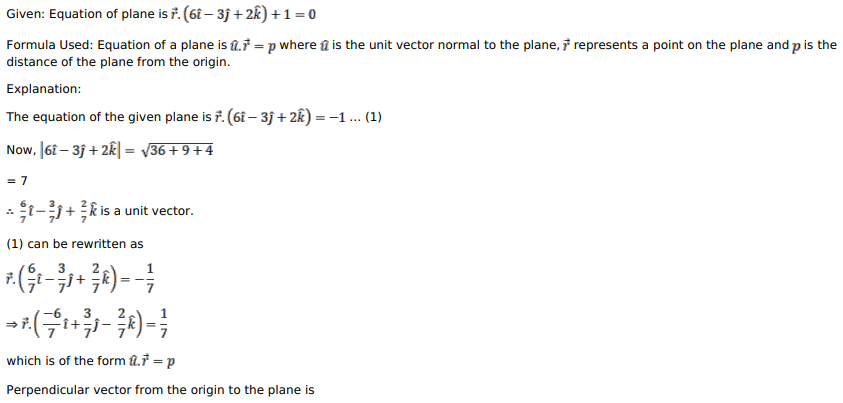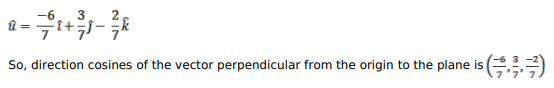# Mark against the correct answer in each of the following:

Question:

Mark against the correct answer in each of the following:

The direction cosines of the perpendicular from the origin to the plane $\overrightarrow{\mathrm{r}} \cdot(6 \hat{\mathrm{i}}-3 \hat{\mathrm{j}}+2 \hat{\mathrm{k}})+1=0$ are

A. $\frac{6}{7}, \frac{3}{7}, \frac{-2}{7}$

B. $\frac{6}{7}, \frac{-3}{7}, \frac{2}{7}$

C. $\frac{-6}{7}, \frac{3}{7}, \frac{2}{7}$

D. None of these

Solution: